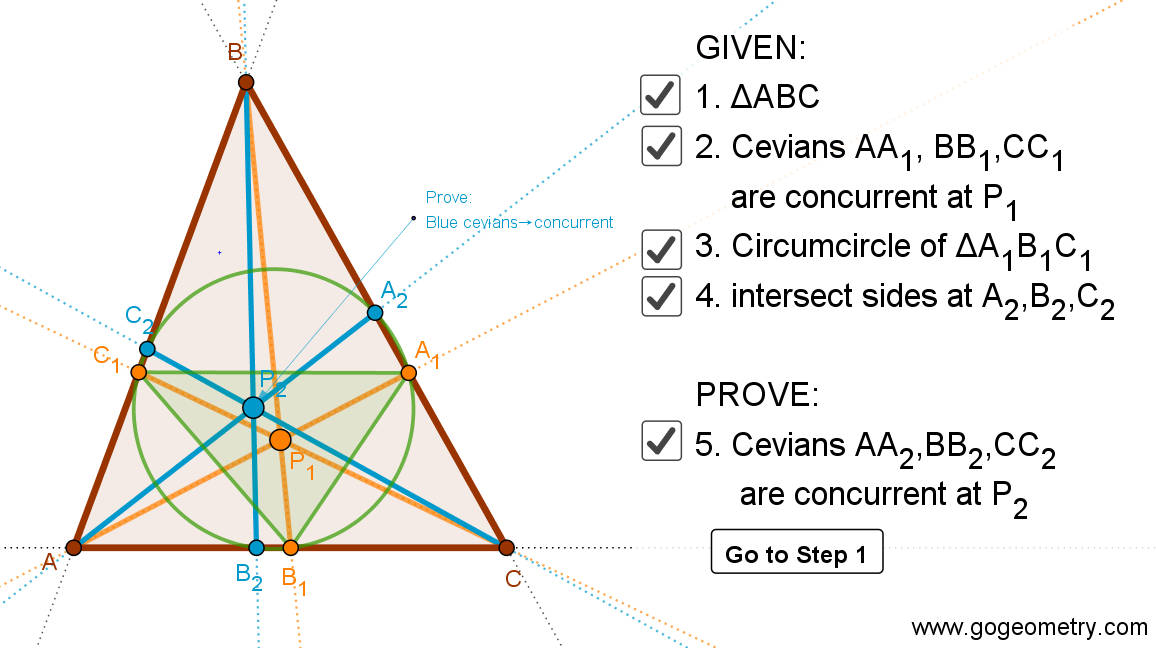# Dynamic Geometry 1478: Reuschle-Terquem Theorem, Concurrent Cevians, Triangle, Circumcircle, Secant line, Cyclocevian, Step-by-step Illustration

In a triangle ABC the cevians AA1,BB1,CC1 are concurrent at P1. The circumcircle of triangle A1B1C1 intercept the sides at A2,B2,C2. Prove that the cevians AA2,BB2,CC2 are concurrent at a point P2 known as cyclocevian conjugate of P1. See dynamic diagram.

Weisstein, Eric W. "Cyclocevian Conjugate." From MathWorld--A Wolfram Web Resource. https://mathworld.wolfram.com/CyclocevianConjugate.html

## Static Diagram of Reuschle-Terquem Theorem## Poster of the Reuschle-Terquem Theorem using iPad Apps### Classroom Resource:Interactive step-by-step animation using GeoGebra

This step-by-step interactive illustration was created with GeoGebra.

• To explore (show / hide): click/tap a check box.
• To stop/play the animation: click/tap the icon in the lower left corner.
• To go to first step: click/tap the "Go to step 1" button.
• To manipulate the interactive figure: click/tap and drag the blue points or figures.

GeoGebra is free and multi-platform dynamic mathematics software for all levels of education that joins geometry, algebra, tables, graphing, statistics and calculus application, intended for teachers and students. Many parts of GeoGebra have been ported to HTML5.